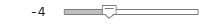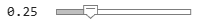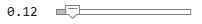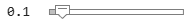designParamEQ

Design parametric equalizer

Description

example

[B,A] = designParamEQ(N,gain,centerFreq,bandwidth) designs an Nth-order parametric equalizer with specified gain, center frequency, and bandwidth. B and A are matrices of numerator and denominator coefficients, with columns corresponding to cascaded second-order section (SOS) filters.

example

[B,A] = designParamEQ(N,gain,centerFreq,bandwidth,mode) specifies whether the parametric equalizer is implemented with second-order sections or fourth-order sections (FOS).

[B,A] = designParamEQ(___,Name,Value) specifies options using one or more Name,Value pair arguments.

Examples

collapse all

Specify the filter order, peak gain in dB, normalized center frequencies, and normalized bandwidth of the bands of your parametric equalizer.

N = [2, ...4];

gain = [6, ...-4];

centerFreq = [0.25, ...0.75];

bandwidth = [0.12, ...0.1];

Generate the filter coefficients using the specified parameters.

[B,A] = designParamEQ(N,gain,centerFreq,bandwidth,"Orientation","row");

fvtool([B,A]);Design a second-order sections (SOS) parametric equalizer using designParamEQ and filter an audio stream.

Create audio file reader and audio device writer System objects. Use the sample rate of the reader as the sample rate of the writer.

frameSize = 256;

deviceWriter = audioDeviceWriter("SampleRate",sampleRate);

Play the audio signal through your device.

count = 0;
while count < 2500
deviceWriter(audio);
count = count + 1;
end

Design an SOS parametric equalizer suitable for use with dsp.BiquadFilter.

N = [4,4];
gain = [-25,35];
centerFreq = [0.01,0.5];
bandwidth = [0.35,0.5];
[B,A] = designParamEQ(N,gain,centerFreq,bandwidth);

Visualize your filter design. Call designParamEQ with the same design specifications. Specify the output orientation as "row" so that it is suitable for use with fvtool.

[Bvisualize,Avisualize] = designParamEQ(N,gain,centerFreq,bandwidth,"Orientation","row");
fvtool([Bvisualize,Avisualize], ...
"FrequencyScale","Log");"SOSMatrixSource","Input port", ...
"ScaleValuesInputPort",false);

Create a spectrum analyzer to visualize the original audio signal and the audio signal passed through your parametric equalizer.

scope = dsp.SpectrumAnalyzer( ...
"SampleRate",sampleRate, ...
"PlotAsTwoSidedSpectrum",false, ...
"FrequencyScale","Log", ...
"FrequencyResolutionMethod","WindowLength", ...
"WindowLength",frameSize, ...
"Title","Original and Equalized Signals", ...
"ShowLegend",true, ...
"ChannelNames",{'Original Signal','Equalized Signal'});

Play the filtered audio signal and visualize the original and filtered spectrums.

count = 0;
while count < 2500
equalizedSignal = myFilter(originalSignal,B,A);
scope([originalSignal(:,1),equalizedSignal(:,1)]);
deviceWriter(equalizedSignal);
count = count + 1;
end

As a best practice, release your objects once done.

release(deviceWriter)
release(scope)Design a fourth-order sections (FOS) parametric equalizer using designParamEQ and filter an audio stream.

Construct audio file reader and audio device writer System objects. Use the sample rate of the reader as the sample rate of the writer.

frameSize = 256;

"RockGuitar-16-44p1-stereo-72secs.wav", ...
"SamplesPerFrame",frameSize);

deviceWriter = audioDeviceWriter( ...
"SampleRate",sampleRate);

Play the audio signal through your device.

count = 0;
while count < 2500
deviceWriter(x);
count = count + 1;
end

Design FOS parametric equalizer coefficients.

N = [2,4];
gain = [5,10];
centerFreq = [0.025,0.65];
bandwidth = [0.025,0.35];
mode = "fos";

[B,A] = designParamEQ(N,gain,centerFreq,bandwidth,mode,"Orientation","row");

Construct FOS IIR filters.

myFilter = dsp.FourthOrderSectionFilter(B,A);

Visualize the frequency response of your parametric equalizer.

fvtool(myFilter)Construct a spectrum analyzer to visualize the original audio signal and the audio signal passed through your parametric equalizer.

scope = dsp.SpectrumAnalyzer( ...
"SampleRate",sampleRate, ...
"PlotAsTwoSidedSpectrum",false, ...
"FrequencyScale","Log", ...
"FrequencyResolutionMethod","WindowLength", ...
"WindowLength",frameSize, ...
"Title","Original and Equalized Signals", ...
"ShowLegend",true, ...
"ChannelNames",{'Original Signal','Equalized Signal'});

Play the filtered audio signal and visualize the original and filtered spectrums.

count = 0;
while count < 2500
y = myFilter(x);

scope([x(:,1),y(:,1)]);

deviceWriter(y);

count = count + 1;
end

As a best practice, release your objects once done.

release(deviceWriter)
release(scope)Input Arguments

collapse all

Filter order, specified as a scalar or row vector the same length as centerFreq. Elements of the vector must be even integers.

Data Types: single | double | int8 | int16 | int32 | int64 | uint8 | uint16 | uint32 | uint64

Peak gain in dB, specified as a scalar or row vector the same length as centerFreq. Elements of the vector must be real-valued.

Data Types: single | double | int8 | int16 | int32 | int64 | uint8 | uint16 | uint32 | uint64

Normalized center frequency of equalizer bands, specified as a scalar or row vector of real values in the range 0 to 1, where 1 corresponds to the Nyquist frequency (π rad/sample). If centerFreq is specified as a row vector, separate equalizers are designed for each element of centerFreq.

Data Types: single | double | int8 | int16 | int32 | int64 | uint8 | uint16 | uint32 | uint64

Normalized bandwidth, specified as a scalar or row vector the same length as centerFreq. Elements of the vector are specified as real values in the range 0 to 1, where 1 corresponds to the Nyquist frequency (π rad/sample).

Normalized bandwidth is measured at gain/2 dB. If gain is set to -Inf (notch filter), normalized bandwidth is measured at the 3 dB attenuation point: $10×{\mathrm{log}}_{10}\left(0.5\right)$.

To convert octave bandwidth to normalized bandwidth, calculate the associated Q-factor as

$Q=\frac{\sqrt{{2}^{\left(octave\text{\hspace{0.17em}}bandwidth\right)}}}{{2}^{\left(octave\text{\hspace{0.17em}}bandwidth\right)}-1}\text{\hspace{0.17em}}.$

Then convert to bandwidth

$bandwidth=\frac{centerFreq}{Q}\text{\hspace{0.17em}}.$

Data Types: single | double | int8 | int16 | int32 | int64 | uint8 | uint16 | uint32 | uint64

Design mode, specified as 'sos' or 'fos'.

• 'fos' –– Implements your equalizer as cascaded fourth-order filters. Because fourth-order sections do not require the computation of roots, they are generally more computationally efficient.

Data Types: char | string

Name-Value Arguments

Specify optional comma-separated pairs of Name,Value arguments. Name is the argument name and Value is the corresponding value. Name must appear inside quotes. You can specify several name and value pair arguments in any order as Name1,Value1,...,NameN,ValueN.

Example: 'Orientation',"row"

Orientation of returned filter coefficients, specified as the comma-separated pair consisting of 'Orientation' and "column" or "row":

Data Types: char | string

Output Arguments

collapse all

Numerator filter coefficients, returned as a matrix. The size and interpretation of B depends on the Orientation and mode:

• If 'Orientation' is set to "column" and mode is set to "sos", then B is returned as an L-by-3 matrix. Each column corresponds to the numerator coefficients of your cascaded second-order sections.

• If 'Orientation' is set to "column" and mode is set to "fos", then B is returned as an L-by-5 matrix. Each column corresponds to the numerator coefficients of your cascaded fourth-order sections.

• If 'Orientation' is set to "row" and mode is set to "sos", then B is returned as a 3-by-L matrix. Each row corresponds to the numerator coefficients of your cascaded second-order sections.

• If 'Orientation' is set to "row" and mode is set to "fos", then B is returned as a 5-by-L matrix. Each row corresponds to the numerator coefficients of your cascaded fourth-order sections.

Denominator filter coefficients, returned as a matrix. The size and interpretation of A depends on the Orientation and mode:

• If 'Orientation' is set to "column" and mode is set to "sos", then A is returned as an L-by-2 matrix. Each column corresponds to the denominator coefficients of your cascaded second-order sections. A does not include the leading unity coefficients.

• If 'Orientation' is set to "column" and mode is set to "fos", then A is returned as an L-by-4 matrix. Each column corresponds to the denominator coefficients of your cascaded fourth-order sections. A does not include the leading unity coefficients.

• If 'Orientation' is set to "row" and mode is set to "sos", then A is returned as a 3-by-L matrix. Each row corresponds to the denominator coefficients of your cascaded second-order sections.

• If 'Orientation' is set to "row" and mode is set to "fos", then A is returned as a 5-by-L matrix. Each row corresponds to the denominator coefficients of your cascaded fourth-order sections.

 Orfanidis, Sophocles J. "High-Order Digital Parametric Equalizer Design." Journal of the Audio Engineering Society. Vol. 53, November 2005, pp. 1026–1046.

Audio Toolbox DocumentationGet trial now